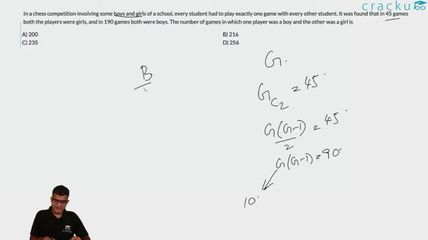Question 10

# In a chess competition involving some boys and girls of a school, every student had to play exactly one game with every other student. It was found that in 45 games both the players were girls, and in 190 games both were boys. The number of games in which one player was a boy and the other was a girl is

Solution

Number of games in which both the players are girls = $$^GC_2$$ where G is the number of girls
$$^GC_2 = 45$$
$$^{10}C_2 = 45$$
So, G = 10
Similarly, number of games in which both the players are boys = $$^BC_2$$, where B is the number of boys
$$^BC_2 = 190$$
$$^{20}C_2 = 190$$
So, B = 20
So, number of games in which one player is a boy and the other player is a girl is 20*10 = 200

### View Video Solution